Next: Unitary Operators Up: Operators Previous: Eigenfunctions and Eigenvalues

### Hermitian Operators

As mentioned previously, the expectation value of an operator
is given by
(55)

and all physical observables are represented by such expectation values. Obviously, the value of a physical observable such as energy or density must be real, so we require <A> to be real. This means that we must have <A> = <A>*, or
(56)

Operatorswhich satisfy this condition are called Hermitian. One can also show that for a Hermitian operator,
(57)

for any two states
and
.

An important property of Hermitian operators is that their eigenvalues are real. We can see this as follows: if we have an eigenfunction ofwith eigenvalue a, i.e.
, then for a Hermitian operator=
(58)
=
= 0

Since
is never negative, we must have either a = a* or
. Sinceis not an acceptable wavefunction, a = a*, so a is real.

Another important property of Hermitian operators is that their eigenvectors are orthogonal (or can be chosen to be so). Suppose that
and
are eigenfunctions ofwith eigenvalues a and b, with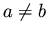. Ifis Hermitian then
=
(59)
=
= 0

since a = a* as shown above. Because we assumed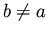, we must have, i.e.andare orthogonal. Thus we have shown that eigenfunctions of a Hermitian operator with different eigenvalues are orthogonal. In the case of degeneracy (more than one eigenfunction with the same eigenvalue), we can choose the eigenfunctions to be orthogonal. We can easily show this for the case of two eigenfunctions ofwith the same eigenvalue. Suppose we have
=
(60)
=
We now want to take linear combinations of
and
to form two new eigenfunctions
and
, where
and
. Now we wantandto be orthogonal, so= 0 (61)
= 0
= 0

Thus we merely need to choose
(62)

and we obtain orthogonal eigenfunctions. This Schmidt-orthogonalization procedure can be extended to the case of n-fold degeneracy, so we have shown that for a Hermitian operator, the eigenvectors can be made orthogonal.Next: Unitary Operators Up: Operators Previous: Eigenfunctions and Eigenvalues